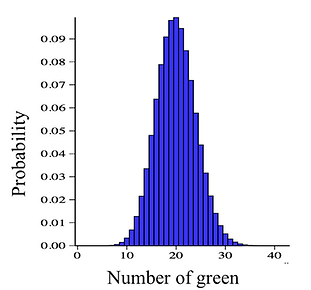## Chapter 6 - Day 8

##### Learning Targets
• Check the 10% condition to be able to assume independence of observations.

• Check the Large Counts condition to us the Normal approximation to the binomial distribution.

##### Activity: Where Are All the Green Skittles?

Up to this point in Section 6.3, we intentionally brushed over a few important details to help students focus on more important ideas (what are the conditions for a binomial distribution, how do we calculate binomial probabilities). Now it is time to address these details.  Specifically, this Activity addresses the 10% condition and the Large Counts condition. These two details are critical for student success when we get to inference, as they will become the conditions necessary to calculate confidence intervals and perform significance tests for proportions.

##### 10% Condition

In the binomial setting, the 10% condition is really about how probabilities change as we sample without replacement. When sampling without replacement, the probability of success for each trial will depend on the outcome of the previous trial(s). But, sometimes this conditional probability changes so little that we can still use the binomial distribution as a model to do probability calculations.

Students will discover this idea in questions #1-4 of the Activity. Question #2 will take them a long time to input into the calculator. They will become angry with you when they complete question #3 and realize they could have used the binomial formula.

##### Large Counts Condition

Under certain conditions, it makes sense to use a Normal distribution to model a binomial distribution. In the Activity, Mr. Wilcox picks 100 Skittles and wants to look at the distribution of possible numbers of green Skittles (p = 0.20). During the debrief, we used this applet to show students what the distribution would look like.Students quickly recognize this as close to a Normal distribution. They also recognize that the answer they got in the Activity using the binomial distribution (#5) is approximately the same as the answer they got using the Normal approximation (#8).

##### So When is the Normal Approximation Good Enough?

During the debrief, we used this applet to explore the conditions necessary for the Normal approximation to be close to the binomial distribution.Help students recognize two ideas:

1. The greater the sample size, the closer the Normal approximation is to the binomial distribution.

2. The closer that p is to 0.5, the more symmetric the binomial distribution, and therefore closer to Normal.

These two ideas are combined to form the Large counts condition np > 10 and n(1 – p) > 10. In other words, the number of successes and the numbers of failures are both at least 10.